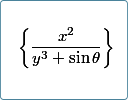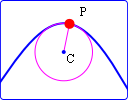Archive for April, 2012

1. Radius of curvature interactive graph
2. Easy math input and nice output using ASCIIMathML and Mathjax
3. Math poll
4. Math puzzles
5. Final thought: Never stop

Easy math input and nice output using ASCIIMathML and MathJaxHere's a system that allows easy input of math (using calculator-like syntax) and good-looking output on any browser.

Radius of Curvature interactive graph updateHere's an updated interactive graph where you can explore the concept of radius of curvature.